# NCERT Solutions class 12 Chemistry Chemical kinetics part 1## myCBSEguide App

CBSE, NCERT, JEE Main, NEET-UG, NDA, Exam Papers, Question Bank, NCERT Solutions, Exemplars, Revision Notes, Free Videos, MCQ Tests & more.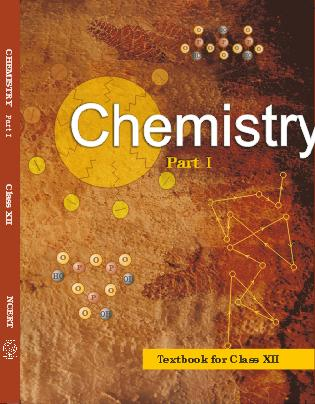## NCERT Class 12 Chemistry Chapter-wise Solutions

•  1 – The Solid State
• 2 – Solutions
• 3 – Electrochemistry
• 4 – Chemical Kinetics
• 5 – Surface Chemistry
• 6 – General Principles and Processes of Isolation of Elements
• 7 – The p-Block Elements
• 8 – The d-and f-Blocks Elements
• 9 – Coordination Compounds
• 10 – Haloalkanes and Haloarenes
• 11 – Alcohols, Phenols and Ethers
• 12 – Aldehydes, Ketones and Carboxylic Acids
• 13 – Amines
• 14 – Biomolecules
• 15 – Polymers
• 16 – Chemistry in Everyday Life

## CHAPTER FOUR CHEMICAL KINETICS

• 4.1 Rate of a Chemical Reaction
• 4.2 Factors Influencing Rate of a Reaction
• 4.3 Integrated Rate Equations
• 4.4 Pseudo First Order Reaction
• 4.5 Temperature Dependence of the rate of a Reaction
• 4.6 Collision Theory of Chemical Reactions

## NCERT Solutions class 12 Chemistry Chemical kinetics part 1

1. From the rate expression for the following reactions, determine their order of reaction and the dimensions of the rate constants.

(i)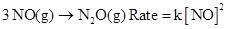(ii)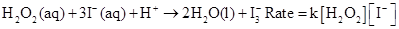(iii)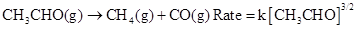(iv)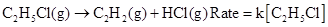2. For the reaction: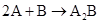the rate =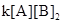with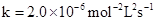. Calculate the initial rate of the reaction when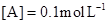,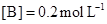. Calculate the rate of reaction after [A] is reduced to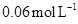.

3. The decomposition of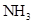on platinum surface is zero order reaction. What are the rates of production of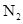and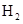if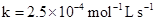?

4. The decomposition of dimethyl ether leads to the formation of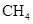,and CO and the reaction rate is given by Rate =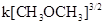. The rate of reaction is followed by increase in pressure in a closed vessel, so the rate can also be expressed in terms of the partial pressure of dimethyl ether, i.e.,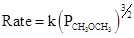If the pressure is measured in bar andtime in minutes, then what are the units of rate and rate constants?

5. Mention the factors that affect the rate of a chemical reaction.

6. A reaction is second order with respect to a reactant. How is the rate of reaction affected if the concentration of the reactant is

(i) doubled

(ii) reduced to half?

7. What is the effect of temperature on the rate constant of a reaction? How can this temperature effect on rate constant be represented quantitatively?

8. In a pseudo first order hydrolysis of ester in water, the following results were obtained: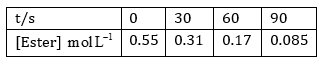(i) Calculate the average rate of reaction between the time interval 30 to 60 seconds.

(ii) Calculate the pseudo first order rate constant for the hydrolysis of ester.

9. A reaction is first order in A and second order in B.

(i) Write the differential rate equation.

(ii) How is the rate affected on increasing the concentration of B three times?

(iii) How is the rate affected when the concentrations of both A and B are doubled?

10. In a reaction between A and B, the initial rate of reaction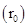was measured for different initial concentrations of A and B as given below: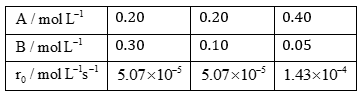What is the order of the reaction with respect to A and B?

11. The following results have been obtained during the kinetic studies of the reaction: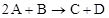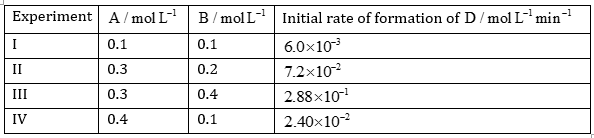Determine the rate law and the rate constant for the reaction.

12. The reaction between A and B is first order with respect to A and zero order with respect to B. Fill in the blanks in the following table: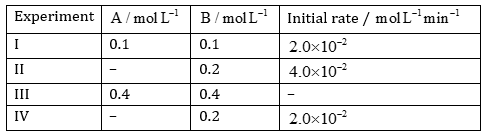13. Calculate the half-life of a first order reaction from their rate constants given below:

(i)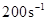(ii)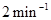(iii) 4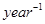14. The half-life for radioactive decay of 14C is 5730 years. An archaeological artifact wood had only 80% of the 14C found in a living tree. Estimate the age of the sample.

15. The experimental data for decomposition of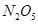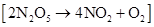in gas phase at 318 K are given below: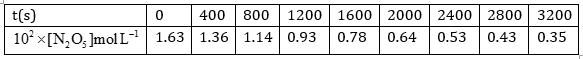(i) Plot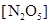against t.

(ii) Find the half-life period for the reaction.

(iii) Draw a graph between logand t.

(iv) What is the rate law?

(v) Calculate the rate constant.

(vi) Calculate the half-life period from k and compare it with (ii).

## NCERT Solutions class 12 Chemistry

NCERT Solutions Class 12 Chemistry PDF (Download) Free from myCBSEguide app and myCBSEguide website. Ncert solution class 12 Chemistry includes text book solutions from both part 1 and part 2. NCERT Solutions for CBSE Class 12 Chemistry have total 16 chapters. 12 Chemistry NCERT Solutions in PDF for free Download on our website. Ncert Chemistry class 12 solutions PDF and Chemistry ncert class 12 PDF solutions with latest modifications and as per the latest CBSE syllabus are only available in myCBSEguide NCERT Solutions class 12 Chemistry Amines part 1

## CBSE app for Class 12

To download NCERT Solutions for class 12 Chemistry, Physics, Biology, History, Political Science, Economics, Geography, Computer Science, Home Science, Accountancy, Business Studies and Home Science; do check myCBSEguide app or website. myCBSEguide provides sample papers with solution, test papers for chapter-wise practice, NCERT solutions, NCERT Exemplar solutions, quick revision notes for ready reference, CBSE guess papers and CBSE important question papers. Sample Paper all are made available through the best app for CBSE students and myCBSEguide website.